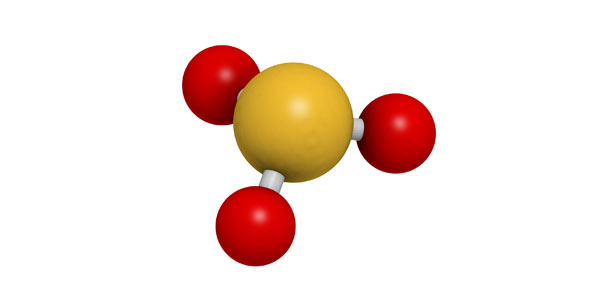# Chemistry: Trivia Questions On Atoms And Elements!

13 Questions | Total Attempts: 51SettingsBelow are some trivia questions on atoms and elements! Elements are made up of atoms that have a specific number of protons and electrons. To calculate the number of atoms in a sample, you need to find how many moles of the element the sample contains. Take up this quiz and get to review what you learned in your chemistry class. All the best!

Related Topics
• 1.
Which drawing represents a correct Bohr's Model?
• A.

A

• B.

B

• C.

C

• 2.
How many neutrons are in tin?
• 3.
How many electrons are in tin?
• 4.
What is the atomic number for tin?
• 5.
How many protons are in tin?
• 6.
What is the atomic mass for tin?
• 7.
Which class of elements is to the left of the zig-zag line on the periodic table of elements?
• A.

Metals

• B.

Non-metals

• C.

Metalloids

• 8.
How many electrons are in fluorine?
• 9.
How many neutrons are in fluorine?
• 10.
What is the atomic number for fluorine?
• 11.
How many protons are in fluorine?
• 12.
What is the atomic mass for fluorine?
• 13.
Which class of element is sulfer (S)?
• A.

Metal

• B.

Non-metal

• C.

Metalloid